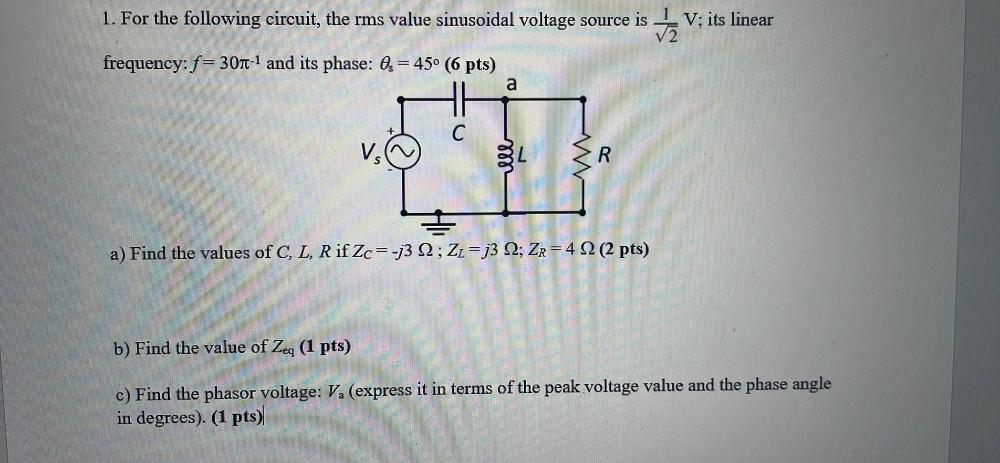Question:

# 1. For the following circuit, the rms value sinusoidal voltage source is DV; its linear frequency: f= 30n-1 and its phase: Os =1. For the following circuit, the rms value sinusoidal voltage source is DV; its linear frequency: f= 30n-1 and its phase: Os = 45° (6 pts) a + C VS ele R a) Find the values of C, L, R if Zc=-j3 2 ; ZŁ= j3 2; Zr= 4 2 (2 pts) b) Find the value of Zeq (1 pts) c) Find the phasor voltage: V. (express it in terms of the peak voltage value and the phase angle in degrees). (1 pts)